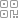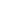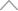5 9 8 1 5 4 1

PyTorch

NumPy

# 相同形状的张量计算

t1 = torch.arange(3)

t1

# tensor([0, 1, 2])

# 对应位置元素相加

t1 + t1

# tensor([0, 2, 4])

### 与Python对比

list

a = 

a + a

# [0, 1, 2, 0, 1, 2]

# 不同形状的张量计算

## 2.1 标量和任意形状的张量

# 标量与一维向量

t1 = torch.arange(3)

# tensor([0, 1, 2])

t1 + 1 # 等效于t1 + torch.tensor(1)

# tensor([1, 2, 3])

# 标量与二维向量

t2 = torch.zeros((34))

t2 + 1 # 等效于t2 + torch.tensor(1)

# tensor([[1., 1., 1., 1.],

#         [1., 1., 1., 1.],

#         [1., 1., 1., 1.]])

## 2.2 相同维度，不同形状张量之间的计算

t2

t2 = torch.zeros(34)

t2

# tensor([[0., 0., 0., 0.],

#         [0., 0., 0., 0.],

#         [0., 0., 0., 0.]])

t21 = torch.ones(14)

t21

# tensor([[1., 1., 1., 1.]])

t21

1×4

t2

3×4

，它们在第一个分量上取值不同，但该分量上

t21

t2 + t21

# tensor([[1., 1., 1., 1.],

#        [1., 1., 1., 1.],

#        [1., 1., 1., 1.]])

t21

t2

t21

1×4

t2

3×4

，然后二者对应位置进行了相加。

t22 = torch.ones(31)

t22

# tensor([[1.],

#         [1.],

#         [1.]])

t2 + t22

# tensor([[1., 1., 1., 1.],

#         [1., 1., 1., 1.],

#         [1., 1., 1., 1.]])

t22+t2

t21+t2

t23 = torch.arange(3).reshape(31)

t23

# tensor([,

#         ,

#         ])

t24 = torch.arange(3).reshape(13)

# tensor([[0, 1, 2]])

t23 + t24

# tensor([[0, 1, 2],

#         [1, 2, 3],

#         [2, 3, 4]])

t23

t24

1×3

，二者的形状在两个份量上均不同，但都有1存在，因此可以广播：t25 = torch.ones(24)

# t2的shape为3×4

t2 + t25

# RuntimeError

## 高维张量的广播

t3 = torch.zeros(234)

t3

# tensor([[[0., 0., 0., 0.],

#          [0., 0., 0., 0.],

#          [0., 0., 0., 0.]],

#         [[0., 0., 0., 0.],

#         [0., 0., 0., 0.],

#         [0., 0., 0., 0.]]])

t31 = torch.ones(231)

t31

# tensor([[[1.],

#          [1.],

#          [1.]],

#         [[1.],

#          [1.],

#          [1.]]])

t3+t31

# tensor([[[1., 1., 1., 1.],

#          [1., 1., 1., 1.],

#          [1., 1., 1., 1.]],

#         [[1., 1., 1., 1.],

#          [1., 1., 1., 1.],

#          [1., 1., 1., 1.]]])

# 不同维度计算中的广播

# 创建一个二维向量

t2 = torch.arange(4).reshape(22)

t2

# tensor([[0, 1],

#         [2, 3]])

# 创建一个三维向量

t3 = torch.zeros(322)

t3

t2 + t3

# tensor([[[0., 1.],

#          [2., 3.]],

#         [[0., 1.],

#          [2., 3.]],

#         [[0., 1.],

#          [2., 3.]]])

t3

t2

1×2×2

3×2×2

• 维度相同时，如果对应分量不同，但有一个为1，就可以广播

• 维度不同时，只需将低维向量的更高维度方向的形状填充为1即可

## 新知精选

IT研发知识▪优秀创作者

### 优选课程 新知学院

•扫码下载商业新知APP~

•回到顶部